# NCERT Solutions for Class 10th Maths Some Applications Of Trigonometry Ex 9.1

In this page we have Class 10 Maths NCERT solutions for Some Applications Of Trigonometry for Exercise 9.1 on pages 203,204 and 205. Hope you like them and do not forget to like , social_share and comment at the end of the page.
Important Definition
(i) The line of sight is the line drawn from the eye of an observer to the point in the object viewed by the observer.
(ii) The angle of elevation of an object viewed, is the angle formed by the line of sight with the horizontal when it is above the horizontal level, i.e., the case when we raise our head to look at the object.
(iii) The angle of depression of an object viewed, is the angle formed by the line of sight with the horizontal when it is below the horizontal level, i.e., the case when we lower our head to look at the object.

## Some Applications Of Trigonometry Exercise 9.1

Question 1
A circus artist is climbing a 20 m long rope, which is tightly stretched and tied from the top of a vertical pole to the ground. Find the height of the pole, if the angle made by the rope with the ground level is 30° (see below figure).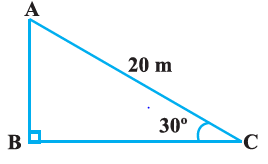Solution
Let AB be the vertical pole AC be 20 m long rope tied to point C.
In ΔABC,
sin 30° = Perpendicular/Base=AB/AC
⇒ 1/2 = AB/20
⇒ AB = 20/2
⇒ AB = 10
The height of the pole is 10 m.

Question 2
A tree breaks due to storm and the broken part bends so that the top of the tree touches the ground making an angle 30° with it. The distance between the foot of the tree to the point where the top touches the ground is 8 m. Find the height of the tree.
Solution
Let us draw the situation belowLet AC be the broken part of the tree.
AB +AC is the tree height
In right ΔABC,
cos 30° = Base/Hypotenuse =BC/AC
⇒ √3/2 = 8/AC
⇒ AC = 16/√3
Also,
tan 30° = AB/BC
⇒ 1/√3 = AB/8
⇒ AB = 8/√3
Total height of the tree = AB+AC = 16/√3 + 8/√3 = 24/√3

Question 3
A contractor plans to install two slides for the children to play in a park. For the children below the age of 5 years, she prefers to have a slide whose top is at a height of 1.5 m, and is inclined at an angle of 30° to the ground, whereas for elder children, she wants to have a steep slide at a height of 3 m, and inclined at an angle of 60° to the ground. What should be the length of the slide in each case?
Solution
Let us take small slide first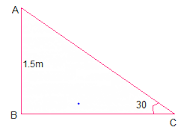AB is the height  and AC is the length of the slide
In right ΔABC,
sin 30° = Perpendicular/hypotenuse=AB/AC
⇒ 1/2 = 1.5/AC
⇒ AC = 3m
Now take the case of elder children slide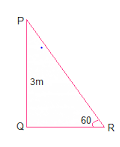PQ is the height
PR is the length of slide
In r ΔPQR,
sin 60° = Perpendicular/hypotenuse= PQ/PR
⇒ √3/2 = 3/PR
⇒ PR = 2√3 m
Hence, length of the slides are 3 m and 2√3 m respectively.

Question 4
The angle of elevation of the top of a tower from a point on the ground, which is 30 m away from the foot of the tower, is 30°. Find the height of the tower.
Solution
The situation is depicted in the below figure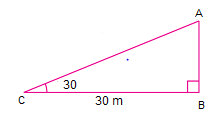AB is height of the tower
BC =30 m (given)
In right ΔABC,
tan 30° = Perpendicular/base=AB/BC
⇒ 1/√3 = AB/30
⇒ AB = 10√3
Thus, the height of the tower is 10√3 m.

Question 5
A kite is flying at a height of 60 m above the ground. The string attached to the kite is temporarily tied to a point on the ground. The inclination of the string with the ground is 60°. Find the length of the string, assuming that there is no slack in the string.
Solution
The situation is depicted in the below figure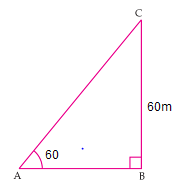Let BC be the height of the kite from the ground,
AC be the inclined length of the string from the ground and A is the point where string of the kite is tied.
In  ΔABC,
sin 60° =Perpendicular/base= BC/AC
⇒ √3/2 = 60/AC
⇒ AC = 40√3 m
Thus, the length of the string from the ground is 40√3 m.

Question 6
A 1.5 m tall boy is standing at some distance from a 30 m tall building. The angle of elevation from his eyes to the top of the building increases from 30° to 60° as he walks towards the building. Find the distance he walked towards the building
Solution
The situation is depicted in the below figure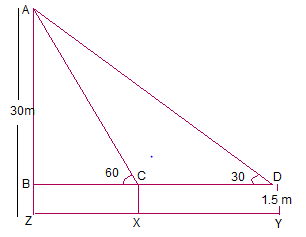Given
DY=1.5m
Let the boy initially standing at point Y with inclination 30° and then he approaches the building to the point X with inclination 60°.
so XY is the distance he walked towards the building.
Height of the building = AZ=30 m
also, XY = CD.
AB = AZ - BZ = (30 - 1.5) = 28.5 m
In right ΔABD,
tan 30° = AB/BD
⇒ 1/√3 = 28.5/BD
⇒ BD = 28.5√3 m
also,
In right ΔABC,
tan 60° = AB/BC
⇒ √3 = 28.5/BC
⇒ BC = 28.5/√3 = 28.5√3/3 m
∴ XY = CD = BD - BC = (28.5√3 - 28.5√3/3) = 28.5√3(1-1/3) = 28.5√3 × 2/3 = 57/√3 m.
Thus, the distance boy walked towards the building is 57/√3 m.

Question 7
From a point on the ground, the angles of elevation of the bottom and the top of a transmission tower fixed at the top of a 20 m high building are 45° and 60° respectively. Find the height of the tower.
Solution
The situation is depicted in the below figure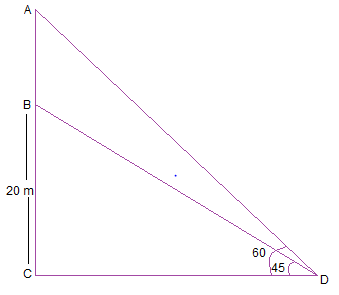D is the point on Ground
BC be the 20 m high building..
Height of transmission tower = AB = AC - BC
In right ΔBCD,
tan 45° = BC/CD
⇒ 1 = 20/CD
⇒ CD = 20 m
also,
In right ΔACD,
tan 60° = AC/CD
⇒ √3 = AC/20
⇒ AC = 20√3 m
Height of transmission tower = AB = AC - BC = (20√3 - 20) m = 20(√3 - 1) m.

Question 8
A statue, 1.6 m tall, stands on the top of a pedestal. From a point on the ground, the angle of elevation of the top of the statue is 60° and from the same point the angle of elevation of the top of the pedestal is 45°. Find the height of the pedestal.
Solution
The situation is depicted in the below figure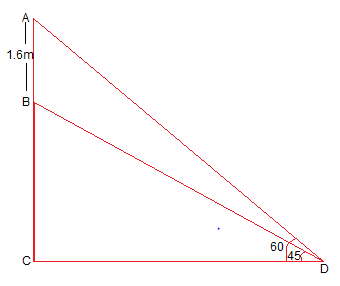Let AB be the height of statue.
D is the point on the ground from where the elevation is taken.
Height of pedestal = BC = AC - AB
In right ΔBCD,
tan 45° = BC/CD
⇒ 1 = BC/CD
⇒ BC = CD.
also,
In right ΔACD,
tan 60° = AC/CD
⇒ √3 = AB+BC/CD
⇒ √3CD = 1.6 m + BC
⇒ √3BC = 1.6 m + BC
⇒ √3BC - BC = 1.6 m
⇒ BC(√3-1) = 1.6 m
⇒ BC = 1.6/(√3-1) m
⇒ BC = 0.8(√3+1) m
Thus, the height of the pedestal is 0.8(√3+1) m.

Question 9
The angle of elevation of the top of a building from the foot of the tower is 30° and the angle of elevation of the top of the tower from the foot of the building is 60°. If the tower is 50 m high, find the height of the building.
Solution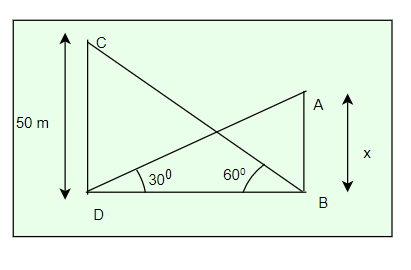Let CD be the tower whose height is equal to 50 m
Let AB be the building and height of the building is x
BC be the distance between the foots of the building and the tower.

In right Δ BCD,
$\tan 60 = \frac {CD}{BC}$
$\sqrt {3} = \frac {50}{BC}$
$BC = \frac {50}{\sqrt {3}}$
Also,
In right Δ ABC,
$\tan 30 = \frac {AB}{BC}$
$\frac {1}{\sqrt {3}} = \frac {x}{BC}$
Substituting the value of BC from above
$x = \frac {50}{3}$ m
Hence the height of the building is 50/3 m.

Question 10
Two poles of equal heights are standing opposite each other on either side of the road, which is 80 m wide. From a point between them on the road, the angles of elevation of the top of the poles are 60° and 30°, respectively. Find the height of the poles and the distances of the point from the poles.
Solution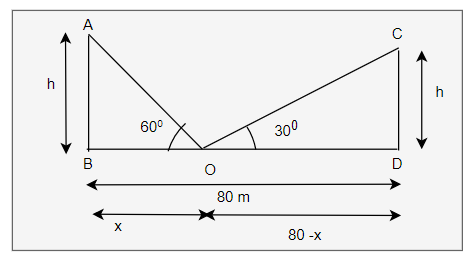Let AB and CD be the two poles of equal height h
O is the point on the road from where elevation of the top of the poles are 60° and 30°, respectively
Let OB=x and OD= 80 -x
Now
In right ΔOAB,
$\tan 60 = \frac {AB}{OB}$
$\sqrt {3} = \frac {h/x}$
or $h = x \sqrt {3}$ --(1)
Also,
In right Δ OCD,
$\tan 30 = \frac {CD}{OB}$
$\frac {1}{\sqrt {3}} = \frac {h}{80-x}$
Substituting the value from h
$\frac {1}{\sqrt {3}} = \frac {x \sqrt {3}}{80-x}$
80-x = 3x
x = 20
Putting the value of x in equation (1)
$h= 20 \sqrt {3}$
Also,
OD= 80 -20 = 60 m
Thus, the height of the poles are $20 \sqrt {3}$ m and distance from the point of elevation are 20 m and 60 m respectively.

Question 11
A TV tower stands vertically on a bank of a canal. From a point on the other bank directly opposite the tower, the angle of elevation of the top of the tower is 60°. From another point 20 m away from this point on the line joing this point to the foot of the tower, the angle of elevation of the top of the tower is 30° . Find the height of the tower and the width of the canal.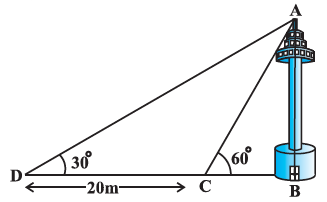Solution
In right Δ ABD,
$\tan 30 = \frac {AB}{BD}$
$\frac {1}{\sqrt {3}} = \frac {AB}{20+BC}$
$AB = \frac {20+BC}{\sqrt {3}}$... (A)
Also,
In right Δ ABC,
$\tan 60 = \frac {AB}{BC}$
$\sqrt {3} = \frac {AB}{BC}$
$AB = \sqrt {3} BC$ ... (B)
From equation (A) and (B)
$\sqrt {3} BC = \frac {20+BC}{\sqrt {3}}$
BC = 10 m
Putting the value of BC in eqn (B)
AB = $10 \sqrt {3}$ m
Hence, the height of the tower $10 \sqrt {3}$ m and the width of the canal is 10 m.

Question 12
From the top of a 7 m high building, the angle of elevation of the top of a cable tower is 60° and the angle of depression of its foot is 45°. Determine the height of the tower.
Solution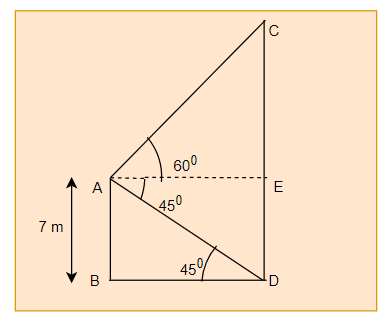Now
Height of Tower = CD = CE + ED= CE + AB

Now
In right Δ AEC,
$\tan 60 =\frac {CE}{AE}$
or
$CE= AE \sqrt {3}$
ALso
In right Δ ABD
$\tan 45 = \frac {AB}{BD}$
or BD = AB = 7 cm
Now AE = BD = 7 cm
So, $CE = 7 \sqrt {3}$
So height of tower
$=7 \sqrt {3} + 7$ m

Question 13
As observed from the top of a 75 m high lighthouse from the sea-level, the angles of depression of two ships are 30° and 45°. If one ship is exactly behind the other on the same side of the lighthouse, find the distance between the two ships.
Solution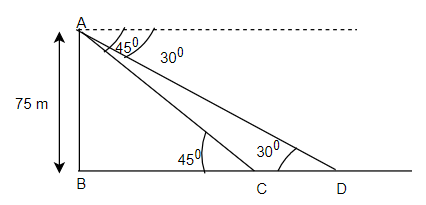Now
In right Δ ABC,
$\tan 45 =\frac {75}{BC}$
or BC = 75 m
ALso
In right Δ ABD,
$\tan 30 = \frac {75}{BC + CD}$
$\frac {1}{\sqrt {3}} = \frac {75}{CD + 75}$
or
CD = $75 (\sqrt {3} -1)$ m

Question 14
A 1.2 m tall girl spots a balloon moving with the wind in a horizontal line at a height of 88.2 m from the ground. The angle of elevation of the balloon from the eyes of the girl at any instant is 60°. After some time, the angle of elevation reduces to 30°. Find the distance travelled by the balloon during the interval.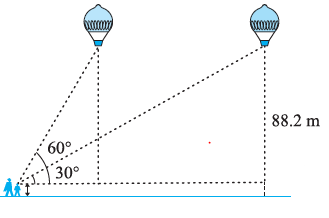Solution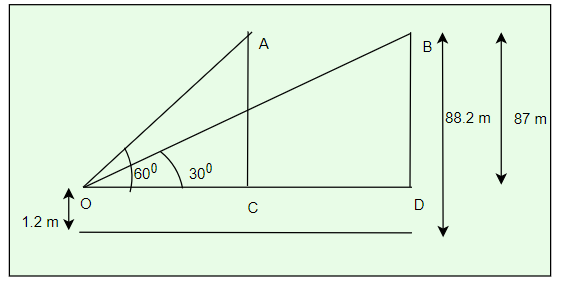Now
In right Δ OAC,
$\tan 60 = \frac {AC}{OC}$
$OC = \frac {87}{\sqrt {3}}$
Also
In right Δ OBD,
$\tan 30 = \frac {BD}{OD}$
$\frac {1}{\sqrt {3}}= \frac {87}{OC + CD}$
$OC + CD= 87 \sqrt {3}$
$CD = 87 \sqrt {3} -\frac {87}{\sqrt {3}}$
$CD = 58 \sqrt {3}$ m

Question 15
A straight highway leads to the foot of a tower. A man standing at the top of the tower observes a car at an angle of depression of 30°, which is approaching the foot of the tower with a uniform speed. Six seconds later, the angle of depression of the car is found to be 60°. Find the time taken by the car to reach the foot of the tower from this point.
Solution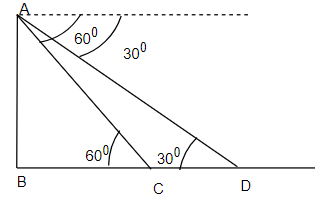In right Δ ABC,
$\tan 60 = \frac {AB}{BC}$
$BC = \frac {AB}{\sqrt {3}}$
Also
In right Δ ABD,
$\tan 30 = \frac {AB}{BD}$
$\frac {1}{\sqrt {3}}=\frac {AB}{BD}$
$BD = AB \sqrt {3}$

Now
$CD = BD - BC = AB \sqrt {3} - \frac {AB}{\sqrt {3}}= \frac {2AB}{\sqrt {3}}$
We can observe that $BC =\frac {1}{2} CD$
Now Time taken to travel is 6 sec,So time taken to travel BC = 6/2 = 3sec

Question 16
The angles of elevation of the top of a tower from two points at a distance of 4 m and 9 m from the base of the tower and in the same straight line with it are complementary. Prove that the height of the tower is 6 m
Solution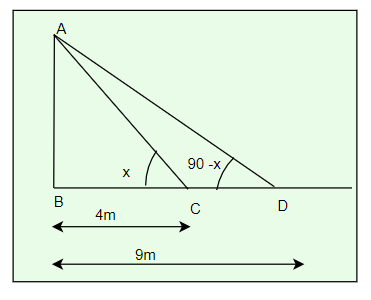Let AB be the tower and C and D are points. Let complementary angles are x and 90 -x
In right Δ ABC,
$\tan x = \frac {AB}{4}$ -(1)

ALso
In right Δ ABD,
$\tan (90-x) = \frac {AB}{9}$
$\cot x = \frac {AB}{9}$
$\tan x = \frac {9}{AB}$ --(2)
From (1) and (2)
$\frac {AB}{4}= \frac {9}{AB}$
$AB^2 = 36$
$AB = 6$

## Summary

1. NCERT Solutions for Class 10th Maths Some Applications Of Trigonometry Exercise 9.1 has been prepared by Expert with utmost care. If you find any mistake.Please do provide feedback on mail. You can download the solutions as PDF in the below Link also
2. This chapter 9 has total 1 Exercise 9.1. This is the only exercise in the chapter.Go back to Class 10 Main Page using below links

### Practice Question

Question 1 What is $1 - \sqrt {3}$ ?
A) Non terminating repeating
B) Non terminating non repeating
C) Terminating
D) None of the above
Question 2 The volume of the largest right circular cone that can be cut out from a cube of edge 4.2 cm is?
A) 19.4 cm3
B) 12 cm3
C) 78.6 cm3
D) 58.2 cm3
Question 3 The sum of the first three terms of an AP is 33. If the product of the first and the third term exceeds the second term by 29, the AP is ?
A) 2 ,21,11
B) 1,10,19
C) -1 ,8,17
D) 2 ,11,20